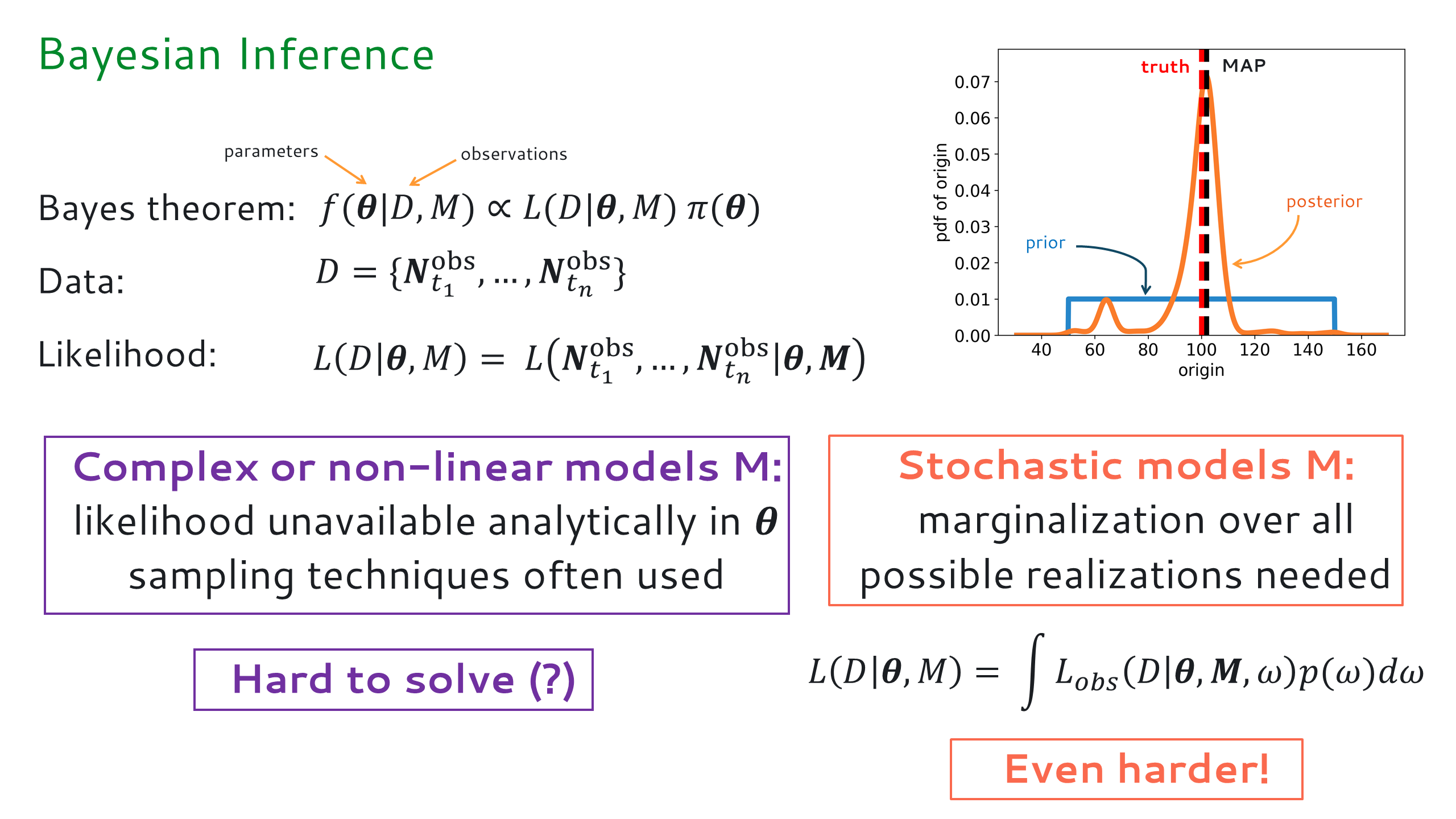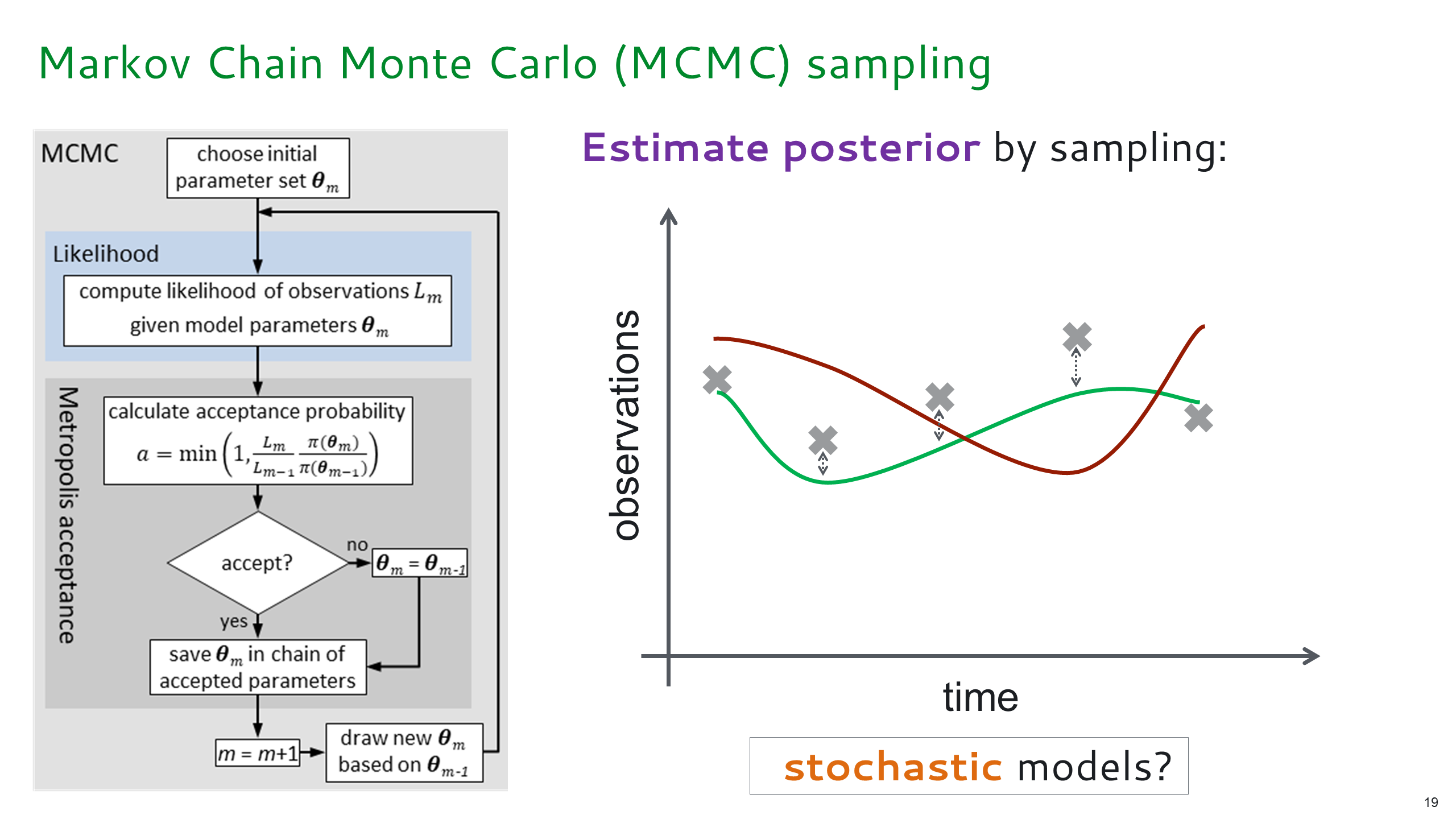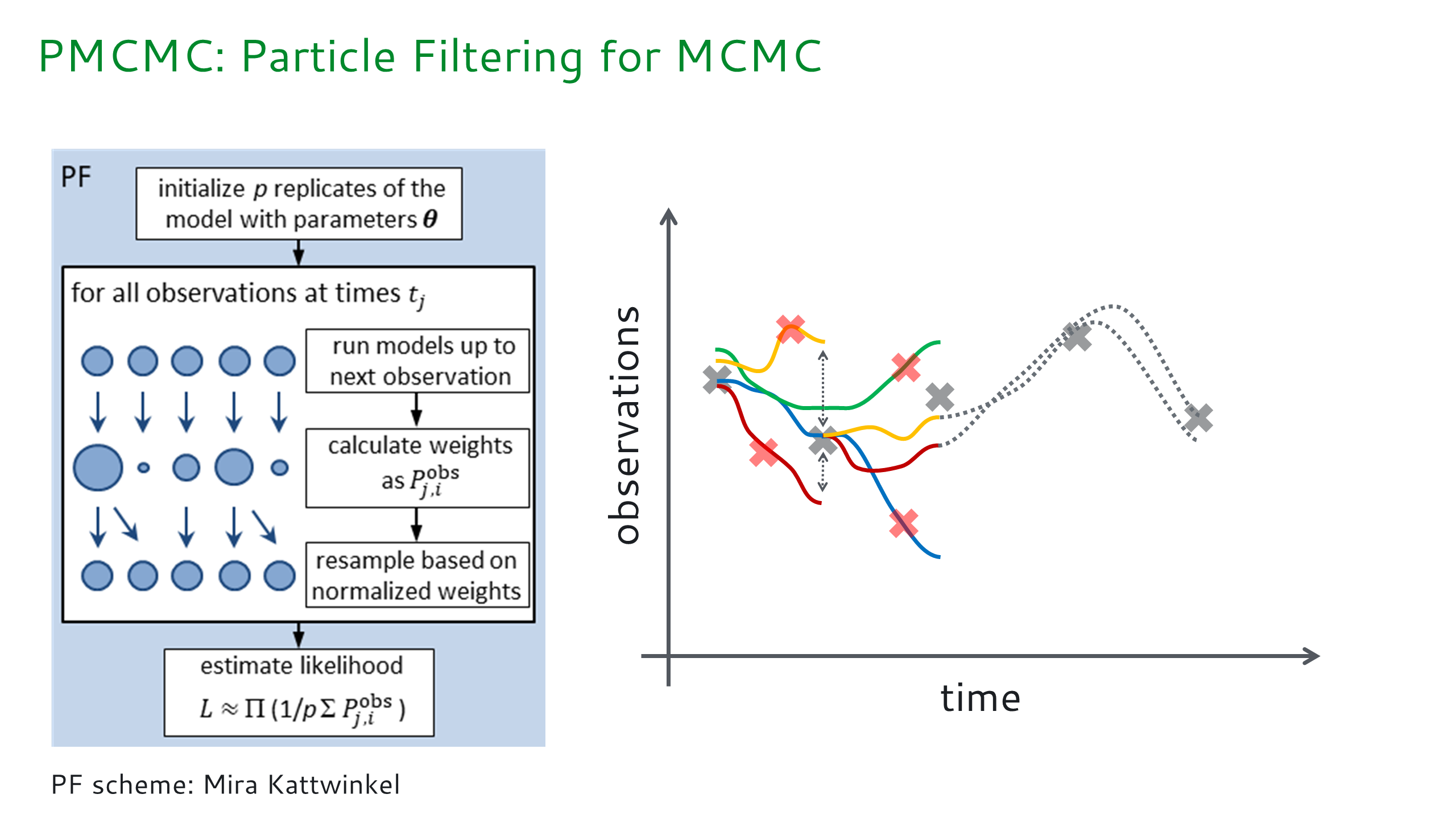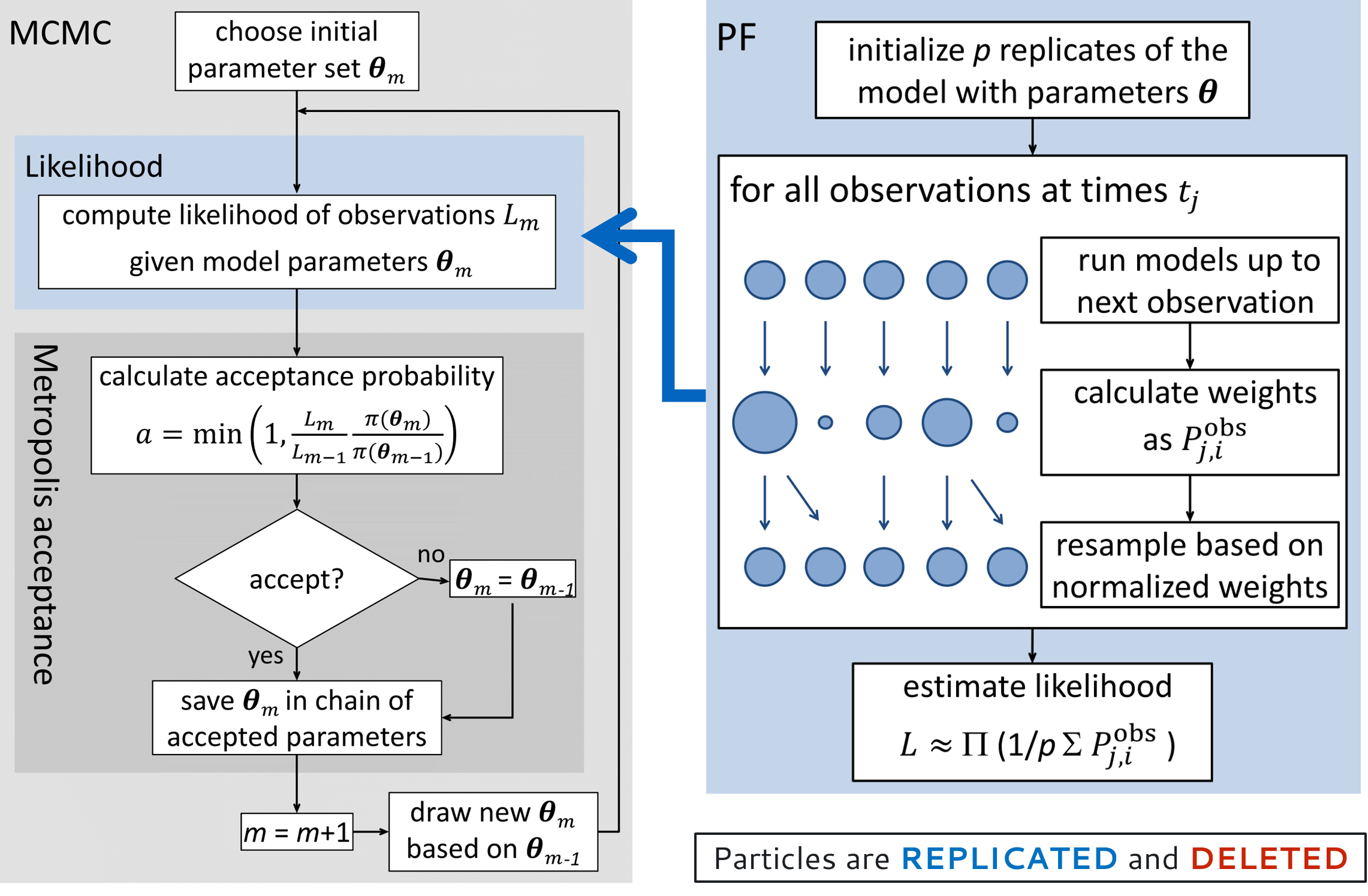# Introduction¶

SPUX stands for “Scalable Package for Uncertainty Quantification”.

## Summary¶

SPUX is a modular framework for Bayesian inference and uncertainty quantification in linear and nonlinear, deterministic and stochastic models. SPUX can be coupled to any model written in any programming language (e.g. Python, R, Julia, C/C++, Fortran, Java). SPUX scales effortlessly from serial runs on a personal computer to parallel high performance computing clusters. SPUX is application agnostic, with current examples available in the field of environmental data sciences.

In the near future, multi-level methods (e.g. ML(ET)PF, MLCV) will be included in SPUX to enable significant algorithmic acceleration of the inference and uncertainty quantification for models that support multiple resolution configurations.

An earlier prototype of spux was already described in a technical paper (preprint available at http://arxiv.org/abs/1711.01410):

```Šukys, J. and Kattwinkel, M.
"SPUX: Scalable Particle Markov Chain Monte Carlo
for uncertainty quantification in stochastic ecological models".
Advances in Parallel Computing - Parallel Computing is Everywhere,
IOS Press, (32), pp. 159–168, 2018.
```

To give you a brief introduction regarding difference aspects of SPUX, we first begin with the mathematical concepts of the underlying scientific problem addressed by this framework.

## Mathematical concepts¶

Here we briefly introduce mathematical concepts used in the Bayesian inference and uncertainty quantification.Bayesian inference: updated the prior distribution of the model parameters to a posterior distribution given model observations (dataset). Bayesian theorem allows us to evaluate (or sample from) the posterior parameter distribution by computing the likelihood of the dataset given candidate model parameters.

## Algorithms¶Markov Chain Monte Carlo (MCMC) for deterministic models M - likelihood of model parameters can be estimated by simply executing the model.Particle Filter (PF) of the stochastic model realizations in order to estimate the likelihood of the model parameters marginalized over all possible scenarios. Note that particles (stochastic model realizations) are adaptively filtered using dataset, making this algorithm very computationally efficient.Markov Chain Monte Carlo (MCMC) and Particle Filtering used in the SPUX framework. Notice, that in most of the real applications using SPUX, an adaptive ensemble affine invariant sampler (EMCEE) with multiple chains is used instead of the conventional single-chain Metropolis-Hastings MCMC.